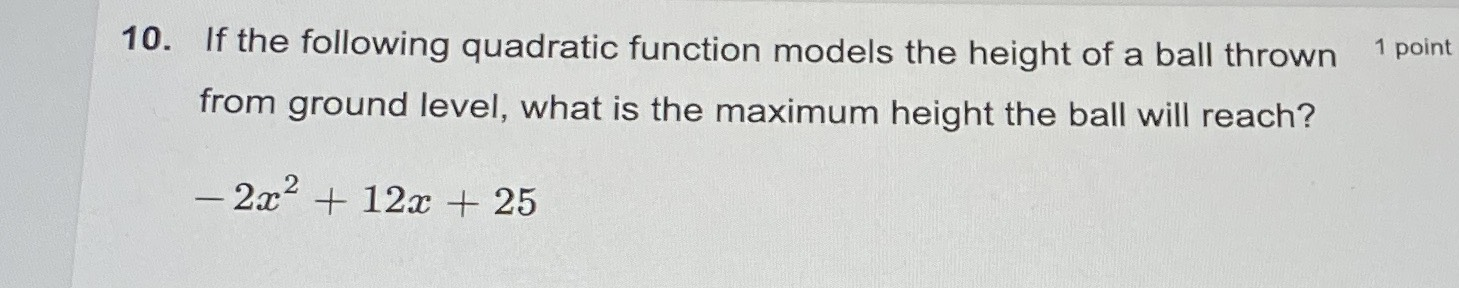### ¿Todavía tienes preguntas de matemáticas?

Pregunte a nuestros tutores expertos
Algebra
Pregunta10. If the following quadratic function models the height of a ball thrown $$1$$ point from ground level, what is the maximum height the ball will reach?

$$- 2 x ^ { 2 } + 12 x + 25$$